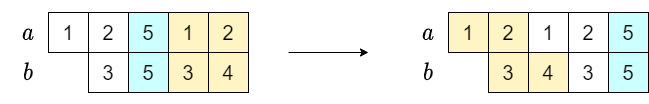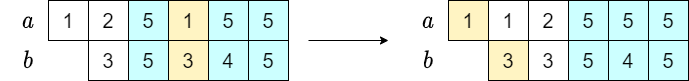Contest Duration: - (local time) (120 minutes) Back to Home
Official

## C - Digit Sum Minimization Editorial by evima

Let $$S(n)$$ denote the sum of the digits of a positive integer $$n$$.

### ◆ The sum of the digits and carries

When adding two integers $$a, b$$, we have $$S(a+b) = S(a)+S(b)$$ if there is no carry.

Since a carry trades $$10$$ in a position for $$1$$ in the position to the left, this operation reduces the sum of the digits by $$9$$. Thus, if there are $$k$$ carries, we have $$S(a+b) = S(a) + S(b) - 9k$$.

The objective of this problem can be rephrased into maximizing the number of carries.

### ◆ The structure of optimal solutions

We can see the following for optimal solutions:

if there is an optimal solution with at least one carry, there is such a solution that has a carry in the ones digit.

Actually, if there is an optimal solution without this property, we can see that it would still be optimal after cycling it so that the first carry happens in the ones digit.Similarly, we can show the following:

if there is an optimal solution with at least $$k$$ carries, there is such a solution that has carries in the $$1$$’s, $$10^1$$’s, $$\ldots$$, $$10^{k-1}$$’s digit.

This can be proved similarly to the above by cycling the part that violates the property.Eventually, solving the following will solve the problem.

Find a permutation $$(a_0,a_1,\ldots)$$ of the digits of $$a$$ and a permutation $$(b_0,b_1,\ldots)$$ of the digits of $$b$$ that makes the following hold for as large $$K$$ as possible.

• $$a_0 + b_0\geq 10$$
• $$a_i + b_i \geq 9$$$$1\leq i < K$$

Here, we assume that $$a_i=0$$ or $$b_i=0$$ if $$i$$ is greater than or equal to the number of digits in $$a$$ or $$b$$.

### ◆ Solution

Since there are just at most $$9$$ possible values for $$a_0$$, it is enough to solve it when $$a_0$$ is fixed.

After fixing $$a_0$$, the following greedy algorithm can construct an optimal solution.

• Choose $$b_0$$ such that $$a_0 + b_0\geq 10$$ as the minimum possible value.
• Choose the pairs $$(a_1,b_1), (a_2,b_2), \ldots$$ in order. When choosing $$b_i$$, choose the minimum among the candidates that have $$a_i+b_i\geq 9$$.

### ◆ Sample Implementation

https://atcoder.jp/contests/arc130/submissions/27440756

posted:
last update: